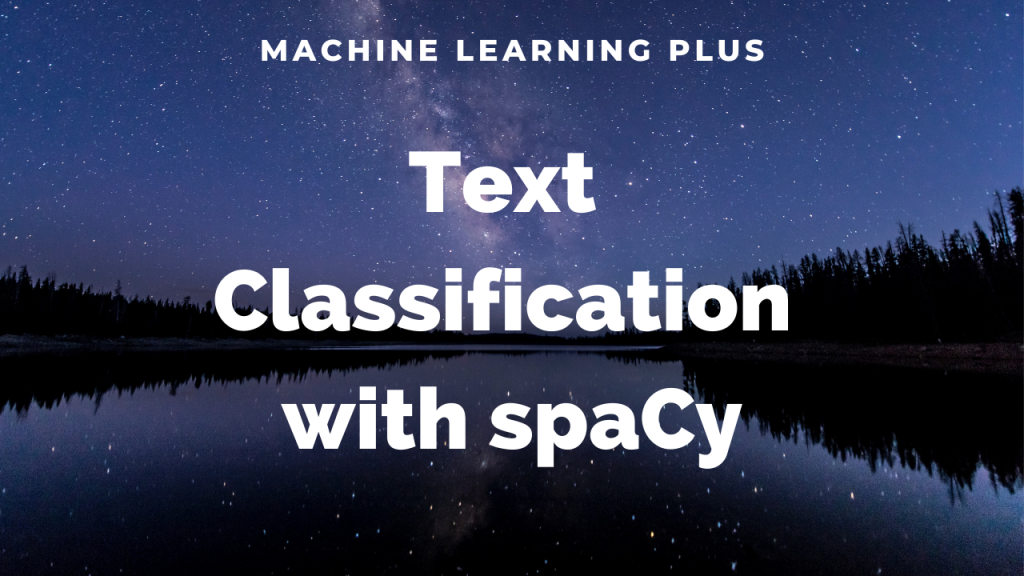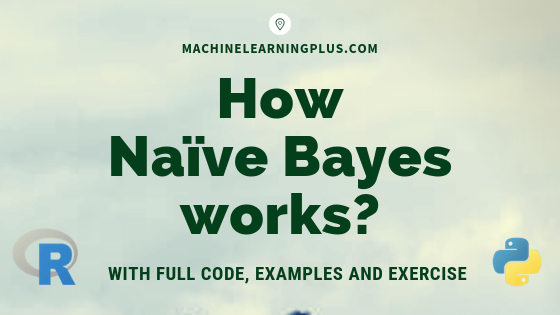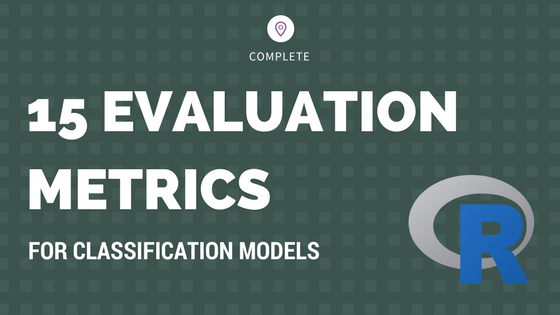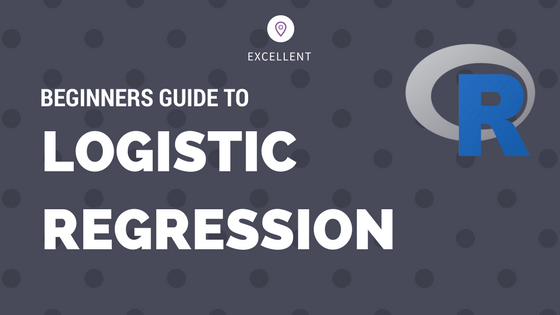Drop a Query

# Classification## Logistic Regression in Julia – Practical Guide with Examples

Julia is a powerful programming language for Machine Learning and Logistic regression is one of the most popular predictive modeling algorithms, used for binary classification. In this one, you will see the full work flow of how to implement churn modeling using Logistic regression in Julia. Logistic Regression with Julia. Photo by Sergio. This is …## SpaCy Text Classification – How to Train Text Classification Model in spaCy (Solved Example)?

Text Classification is the process categorizing texts into different groups. SpaCy makes custom text classification structured and convenient through the textcat component. Text classification is often used in situations like segregating movie reviews, hotel reviews, news data, primary topic of the text, classifying customer support emails based on complaint type etc. For many real-life cases, …## How Naive Bayes Algorithm Works? (with example and full code)

Naive Bayes is a probabilistic machine learning algorithm based on the Bayes Theorem, used in a wide variety of classification tasks. In this post, you will gain a clear and complete understanding of the Naive Bayes algorithm and all necessary concepts so that there is no room for doubts or gap in understanding. Contents 1. …## Caret Package – A Practical Guide to Machine Learning in R

Caret Package is a comprehensive framework for building machine learning models in R. In this tutorial, I explain nearly all the core features of the caret package and walk you through the step-by-step process of building predictive models. Be it a decision tree or xgboost, caret helps to find the optimal model in the shortest …## Evaluation Metrics for Classification Models – How to measure performance of machine learning models?

Choosing the right evaluation metric for classification models is important to the success of a machine learning app. Monitoring only the ‘accuracy score’ gives an incomplete picture of your model’s performance and can impact the effectiveness. So, consider the following 15 evaluation metrics before you finalize on the KPIs of your classifier model. Introduction: Building …## Logistic Regression – A Complete Tutorial With Examples in R

Logistic regression is a predictive modelling algorithm that is used when the Y variable is binary categorical. That is, it can take only two values like 1 or 0. The goal is to determine a mathematical equation that can be used to predict the probability of event 1. Once the equation is established, it can …Course Preview

## Machine Learning A-Z™: Hands-On Python & R In Data Science

### Free Sample Videos:#### Machine Learning A-Z™: Hands-On Python & R In Data Science#### Machine Learning A-Z™: Hands-On Python & R In Data Science#### Machine Learning A-Z™: Hands-On Python & R In Data Science#### Machine Learning A-Z™: Hands-On Python & R In Data Science